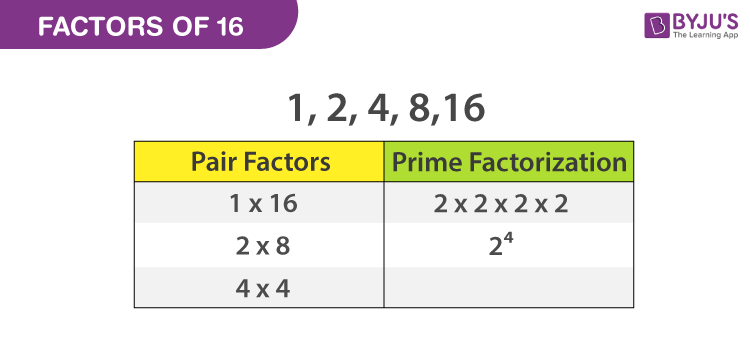# Factors of 16

The factors of 16 are the numbers that produce the result as 16 when two numbers are multiplied together. For example, the pair factors of 16 are written as (1,16) and (-1,-16). When we multiply the pair of negative factors, the result should give the original number, such as multiplying -1  × -16, we get 16. To find the factors of a number, 16, we will use the factorization method.In the article, we are going to learn the pair factors and the prime factors of 16 with complete explanation.

## What are the Factors of 16?

The factors of 16 are the numbers that divide the number 16 completely without leaving any remainder. As the number 16 is a composite number, it has more than one factor. The factors of 16 are 1, 2, 4, 8 and 16. Similarly, the negative factors of 16 are -1, -2, -4, -8 and -16.

 Factors of 16: 1, 2, 4, 8 and 16. Prime Factorization of 16: 2 × 2 × 2 × 2 or 24

## Pair Factors of 16

To find the pair factors of 16, multiply the two numbers in a pair to get the original number as 16, such numbers are as follows

 Positive Pair Factors Negative Pair Factors 1 × 16 = 16 ⇒ (1, 16) -1 × -16 = 16 ⇒ (-1, -16) 2 × 8 = 16 ⇒ (2, 8) -2 × -8 = 16 ⇒ (-2, -8) 4 × 4 = 16 ⇒ (4, 4) -4 × -4 = 16 ⇒ (-4, -4)

## How to calculate the Factors of 16?

Go through the following steps to calculate the factors of 16.

• First, write the number 16.
• Find the two numbers, which gives the result as 16 under the multiplication, say 2 and 8, such as 2 × 8 = 16.
• We know that 2 is a prime number that has only two factors, i.e., 1 and the number itself (1 and 2). So, it cannot be further factorized.
• Look at the number 8, which is a composite number but not a prime number. So it can be further factorized.
• 8 can be factored as 2 x 2 x 2 x 1.
• Therefore, the factorization of 16 is written as, 16 = 2 × 2 × 2 × 2 x 1.

## Prime Factors of 16 By Division Method

The number 16 is a composite and it must have prime factors. Now let us know how to calculate the prime factors of 16.

• The first step is to divide the number 16 with the smallest prime number, say 2.
• 16 ÷ 2 = 8
• Again divide 8 by 2 and the process goes on.
• 8 ÷ 2 = 4
• 4 ÷ 2 = 2
• 2 ÷ 2 = 1

Finally, we received the number 1 at the end of the division process. So that we cannot proceed further. So, the prime factors of 16 are written as 2 × 2 × 2 x 2 or 24, where 2 is a prime number.

## Video Lesson on Prime FactorsRegister with BYJU’S to know more about factors and prime factors of other numbers and also download BYJU’S – The Learning App for a better experience.

 Links Related to Factors Factors of 15 Factor of 36 Factors of 48 Factors of 18 Factors of 24 Factors of 25 Factors of 42 Factors of 60 Factors of 35 Factors of 81 Factors of 75 Factors of 56

### Examples

Example 1:

Find the common factor of 16 and 15.

Solution:

The factors of 16 are 1, 2, 4, 8 and 16.

The factors of 15 are 1, 3, 5 and 15.

The common factor of 15 and 16 is 1.

Example 2:

What is the sum of factors of 16?

Solution:

We know that the factors of 16 are 1, 2, 4, 8 and 16.

Hence, the sum of factors of 16 is 1+2+4+8+16.

Sum of factors of 16 = 31

Example 3:

Find the common factors of 16 and 14.

Solution:

Factors of 16: 1, 2, 4, 8 and 16

Factors of 14: 1, 2, 7 and 14.

Hence, the common factors of 14 and 16 are 1 and 2.

## Frequently Asked Questions on Factors of 16

Q1

### What are the multiples of 16?

The list of multiples of 16 is given by:
16, 32, 48, 64, 80, 96, 112, 128, 144, 160, etc.
Q2

### Are all factors of 16 even?

Except 1, all other factors of 16 are even numbers. Thus, the factors of 16 are 1, 2, 4, 8, 16.
Q3

### What are the prime factors for 16?

The prime factors of 16 as a product can be written as 2 × 2 × 2 x 2 or 2^4, where 2 is the prime number.
Q4

### What are the common factors of 12 and 16?

The common factors are 1, 2, 4 since the factors of 12 are 1, 2, 3, 4, 6, and 12, whereas the factors of 16 are 1, 2, 4, 8, and 16.
Q5

### Is 16 a composite number?

Yes, 16 is a composite number since 16 has more than two factors.
Quiz on Factors of 16